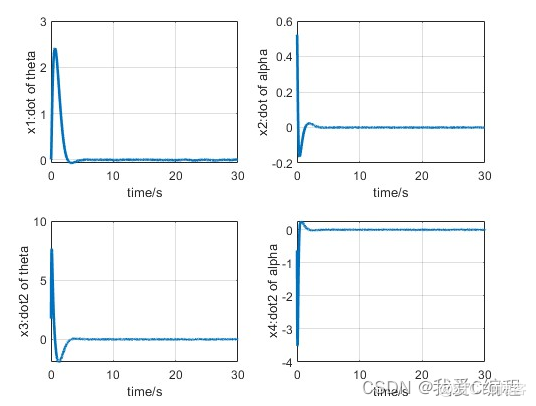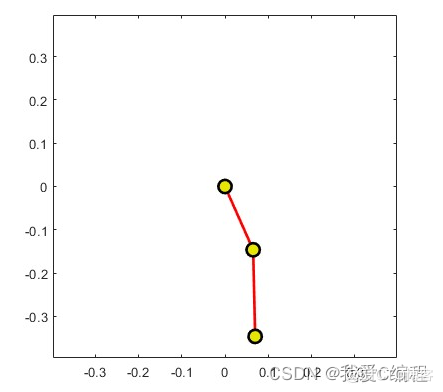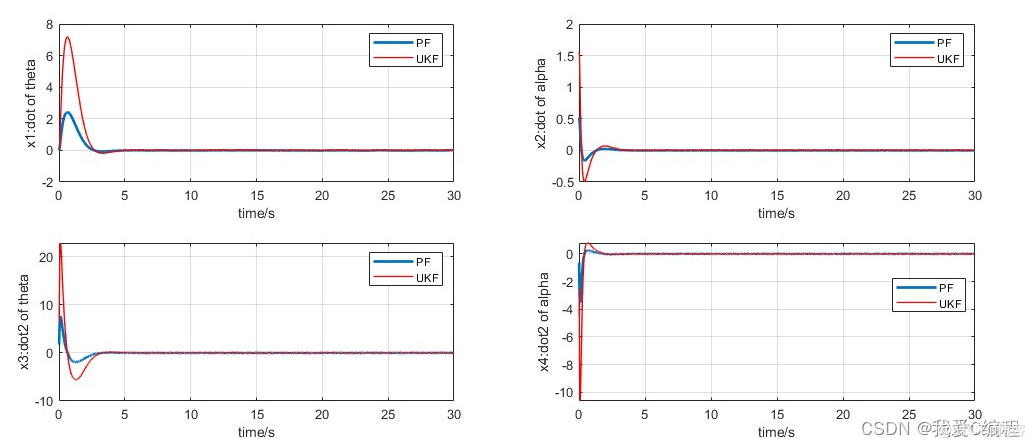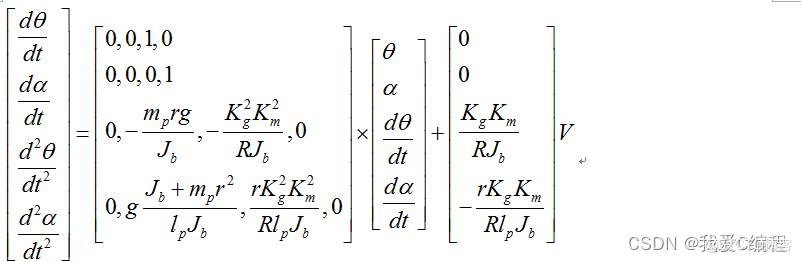# m基于UKF控制器的倒立摆控制系统matlab仿真

+关注继续查看

1.算法仿真效果
matlab2022a仿真结果如下：2.算法涉及理论知识概要

     倒立摆，Inverted Pendulum ，是典型的多变量、高阶次 ，非线性、强耦合、自然不稳定系统。倒立摆系统的稳定控制是控制理论中的典型问题 ，在倒立摆的控制过程中能有效反映控制理论中的许多关键问题 ，如非线性问题、鲁棒性问题、随动问题、镇定、跟踪问题等。因此倒立摆系统作为控制理论教学与科研中典型的物理模型 ，常被用来检验新的控制理论和算法的正确性及其在实际应用中的有效性。从 20 世纪 60 年代开始 ，各国的专家学者对倒立摆系统进行了不懈的研究和探索。
倒立摆控制系统是一个复杂的、不稳定的、非线性系统，是进行控制理论教学及开展各种控制实验的理想实验平台。对倒立摆系统的研究能有效的反映控制中的许多典型问题：如非线性问题、鲁棒性问题、镇定问题、随动问题以及跟踪问题等。通过对倒立摆的控制，用来检验新的控制方法是否有较强的处理非线性和不稳定性问题的能力。同时，其控制方法在军工、航天、机器人和一般工业过程领域中都有着广泛的用途，如机器人行走过程中的平衡控制、火箭发射中的垂直度控制和卫星飞行中的姿态控制等。
本课程，我们的倒立摆模型如下：3.MATLAB核心程序
global Jb;
global lp;
global mp;
global g;

LEN= 10000;
%离散
ts = 0.01;
mp = 0.17;
r = 0.16;
Jb = 0.0024;
R = 8.3;
Km = 0.023;
Kg = 120/16;
lp = 0.2;
g = 9.8;

%根据
a1 = -mprg/Jb;
a2 = -Kg^2Km^2/R/Jb;
a3 = g
(Jb + mpr^2)/lp/Jb;
a4 = r
KgKgKmKm/R/lp/Jb;
b1 = Kg
Km/R/Jb;
b2 = -rKgKm/R/lp/Jb;

A = [0,0 ,1 ,0;
0,0 ,0 ,1;
0,a1,a2,0;
0,a3,a4,0];

B = [0;
0
b1;
b2];

[F,G] = c2d(A,B,ts);
Q = diag([1 1 0 0]);
R = ;
[K,p,e] = dlqr(F,G,Q,R);
F = F-G*K;

%初始条件
x1_0 = 0;
x2_0 = pi/2;
x3_0 = 0;
x4_0 = pi/2;
u_0 = 10;

for k=1:1:LEN
k
.........................................................................
%PF
X(k) = func_ukf_filter(y);
u_0 = X(k);
x1_0 = x1(k);
x2_0 = x2(k);
x3_0 = x3(k);
x4_0 = x4(k);
end|
2天前
|

22 9
|
4天前
|

7 0
|
4天前
|

25 8
|
4天前
|

18 5
|
4天前
|

20 0
|
4天前
|

129 1
|
4天前
|

27 4
|
4天前
|

23 0
|
4天前
|

m基于LTE的通信链路matlab仿真,上行为SC-FDMA和下行为OFDMA
m基于LTE的通信链路matlab仿真,上行为SC-FDMA和下行为OFDMA
19 3
|
4天前
|

m基于Faster-RCNN网络的猫脸检测和猫眼定位系统matlab仿真,带GUI界面
m基于Faster-RCNN网络的猫脸检测和猫眼定位系统matlab仿真,带GUI界面
18 3